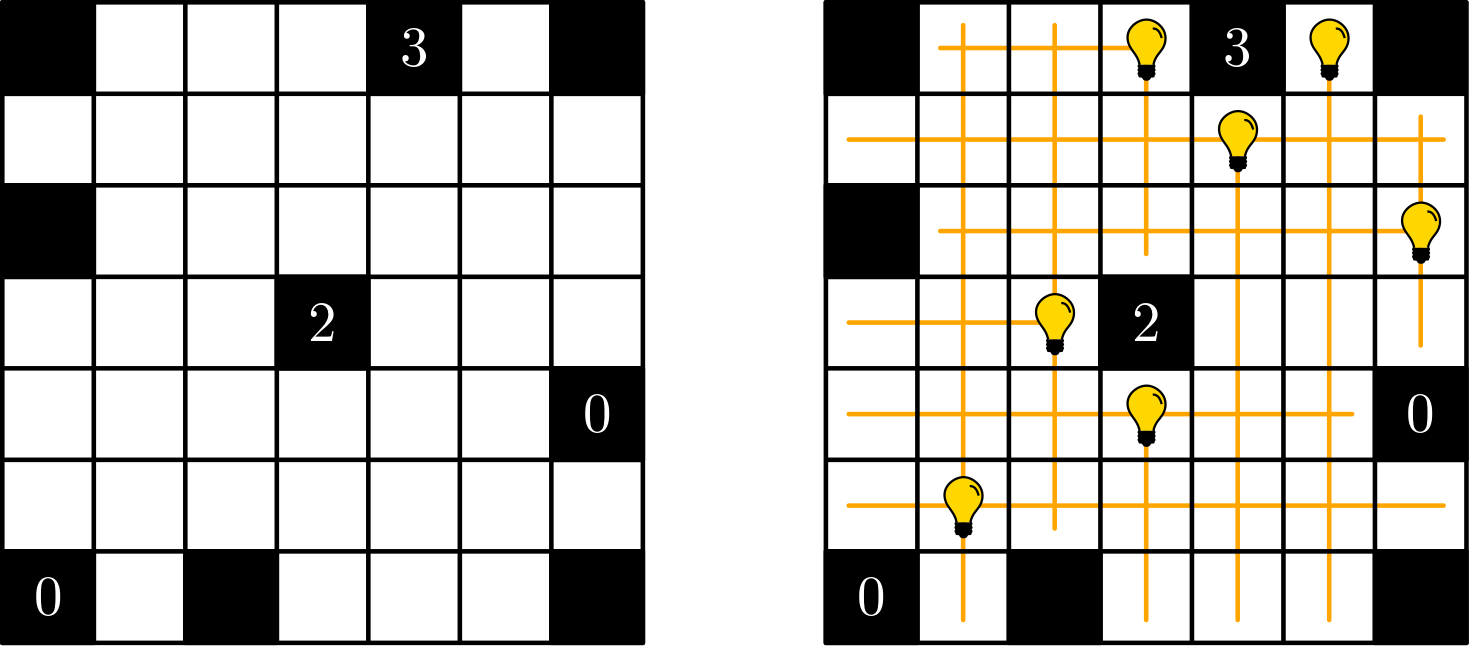# Light Up (Akari)

A single player game in which the player places light bulbs in rectangular black and white grid to light up all white cells.## Description

Light Up is a single player game played on a rectangular grid. Initially, the cells of the grid are either white or black, where black cells can optionally be labeled with an integer between $0$ and $4$ (inclusive).

The player can place light bulbs into white cells. Specifically, a light bulb $b$ lights a white cell $c$ if $b$ lies on the same row or column as $c$ (or both, i.e., $b$ is placed on $c$) and the portion of the row/column between by $b$ and $c$ contains no black cells. The goal is to find an arrangement of light bulbs such that:

• All white cells are lit by at least one light bulb.
• If a white cell $c$ contains a light bulb $b$, then $c$ is lit only by $b$.
• If black cell $c$ is labeled with $\ell$, then the neighboring cells of $c$ (w.r.t. $4$-adjacency) contain exactly $\ell$ light bulbs.

The figure shows an instance of Light Up (left) and a possible solution (right).

## Computational complexity

The problem of deciding whether an instance of Light Up admits a solution is NP-Complete .B. McPhail, “Light Up is NP-complete”.

@misc{cog:light-up,
author = "{CoG contributors}",
title  = "{Light Up (Akari) --- Complexity of Games}",
year   = "2022",
url    = "https://www.isnphard.com/i/light-up/",
note   = "[Online; accessed 2022-06-02]"
}